Test: Integral Calculus

# Test: Integral Calculus

Test Description

## 20 Questions MCQ Test GATE Electrical Engineering (EE) 2023 Mock Test Series | Test: Integral Calculus

Test: Integral Calculus for Electronics and Communication Engineering (ECE) 2023 is part of GATE Electrical Engineering (EE) 2023 Mock Test Series preparation. The Test: Integral Calculus questions and answers have been prepared according to the Electronics and Communication Engineering (ECE) exam syllabus.The Test: Integral Calculus MCQs are made for Electronics and Communication Engineering (ECE) 2023 Exam. Find important definitions, questions, notes, meanings, examples, exercises, MCQs and online tests for Test: Integral Calculus below.
Solutions of Test: Integral Calculus questions in English are available as part of our GATE Electrical Engineering (EE) 2023 Mock Test Series for Electronics and Communication Engineering (ECE) & Test: Integral Calculus solutions in Hindi for GATE Electrical Engineering (EE) 2023 Mock Test Series course. Download more important topics, notes, lectures and mock test series for Electronics and Communication Engineering (ECE) Exam by signing up for free. Attempt Test: Integral Calculus | 20 questions in 60 minutes | Mock test for Electronics and Communication Engineering (ECE) preparation | Free important questions MCQ to study GATE Electrical Engineering (EE) 2023 Mock Test Series for Electronics and Communication Engineering (ECE) Exam | Download free PDF with solutions
 1 Crore+ students have signed up on EduRev. Have you?
Test: Integral Calculus - Question 1

###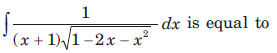​ ​ ​

Detailed Solution for Test: Integral Calculus - Question 1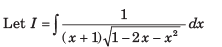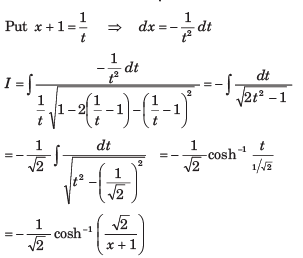Test: Integral Calculus - Question 2

###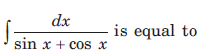​ ​ ​

Detailed Solution for Test: Integral Calculus - Question 2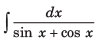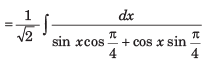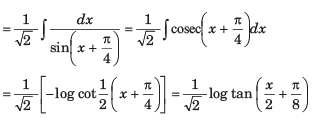Test: Integral Calculus - Question 3

###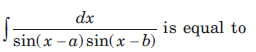Detailed Solution for Test: Integral Calculus - Question 3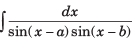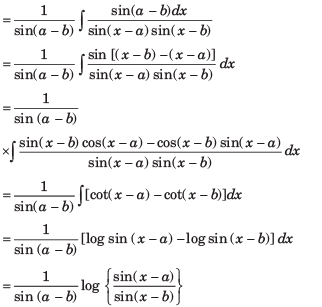Test: Integral Calculus - Question 4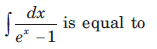Detailed Solution for Test: Integral Calculus - Question 4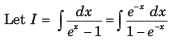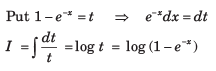Test: Integral Calculus - Question 5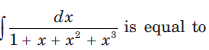Detailed Solution for Test: Integral Calculus - Question 5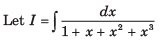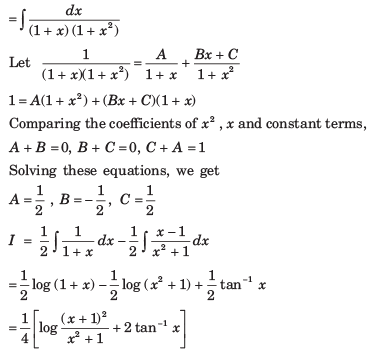Test: Integral Calculus - Question 6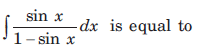Detailed Solution for Test: Integral Calculus - Question 6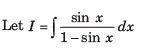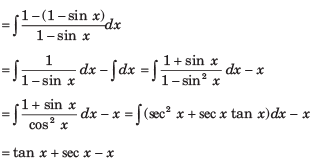Test: Integral Calculus - Question 7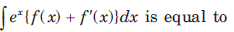Detailed Solution for Test: Integral Calculus - Question 7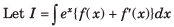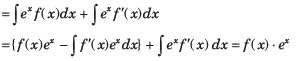Test: Integral Calculus - Question 8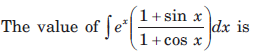Detailed Solution for Test: Integral Calculus - Question 8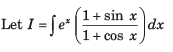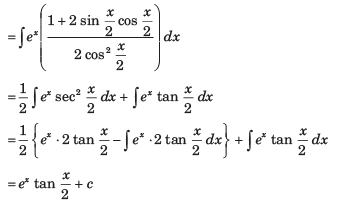Test: Integral Calculus - Question 9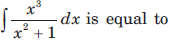Detailed Solution for Test: Integral Calculus - Question 9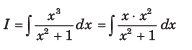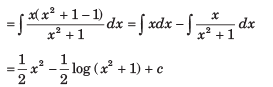Test: Integral Calculus - Question 10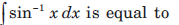Detailed Solution for Test: Integral Calculus - Question 10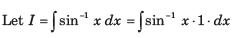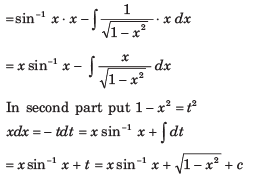Test: Integral Calculus - Question 11

If A is the region bounded by the parabolas y2 = 4x and x2 = 4y then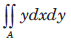is equal to

Detailed Solution for Test: Integral Calculus - Question 11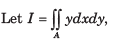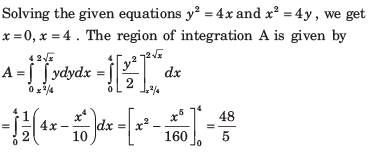Test: Integral Calculus - Question 12

The area of the region bounded by the curves x2 + y2 = a2  and x + y = a in the first quadrant is given by

Detailed Solution for Test: Integral Calculus - Question 12

The curves are

x2 + y2 = a2 ...(i)

and x + y = a ...(ii)

The curves (i) and (ii) intersect at A (a, 0) and B (0,a)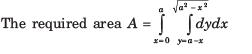Test: Integral Calculus - Question 13

The area bounded by the curves y = 2√x , y = -x , x = 1  and x = 4 is given by

Detailed Solution for Test: Integral Calculus - Question 13

The given equations of the curves are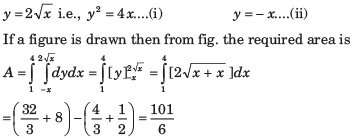Test: Integral Calculus - Question 14

The area bounded by the curves y2 = 9x , x - y + 2 = 0 is given by

Detailed Solution for Test: Integral Calculus - Question 14

The equations of the given curves are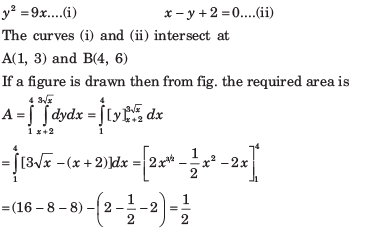Test: Integral Calculus - Question 15

The area of the cardioid r = a (1 + cos θ) is given by

Detailed Solution for Test: Integral Calculus - Question 15

The equation of the cardioid is

r = a (1 + cos θ)  .... (i)

If a figure is drawn then from fig. the required area is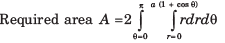Test: Integral Calculus - Question 16

The area bounded by the curve r = θ cosθ and the lines θ = 0 and θ = π/2 is given by

Detailed Solution for Test: Integral Calculus - Question 16

The equation of the given curve is

r = θ cosθ ...(i)

The required area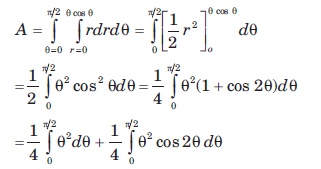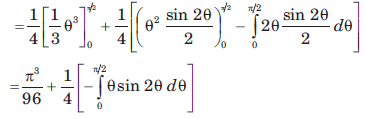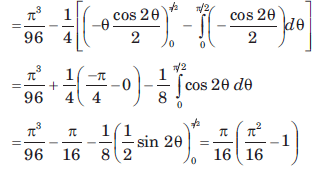Test: Integral Calculus - Question 17

The area of the lemniscate r2 = a2 cos2θ is given by

Detailed Solution for Test: Integral Calculus - Question 17

If a figure is drawn then from fig. the required area is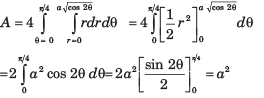Test: Integral Calculus - Question 18

The area of the region bounded by the curve y(x2 + 2) = 3x and 4y = x2 is given by

Detailed Solution for Test: Integral Calculus - Question 18

The equations of given curves are

y(x2 + 2) = 3x ....(i) and 4y = x2 ....(ii)

The curve (i) and (ii) intersect at A (2, 1).
If a figure is drawn then from fig. the required area is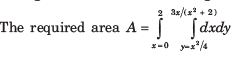Test: Integral Calculus - Question 19

The volume of the cylinder x2 + y2 = a2 bounded below by z = 0 and bounded above by z = h is given by

Detailed Solution for Test: Integral Calculus - Question 19

The equation of the cylinder is  x2 + y2 = a2

The equation of surface CDE is z = h

If a figure is drawn then from fig. the required area is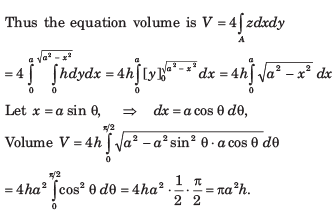Test: Integral Calculus - Question 20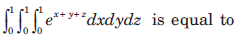Detailed Solution for Test: Integral Calculus - Question 20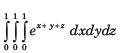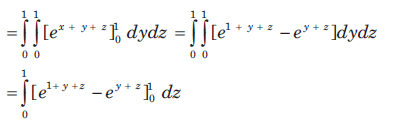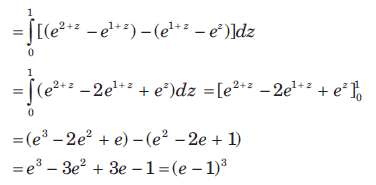## GATE Electrical Engineering (EE) 2023 Mock Test Series

22 docs|274 tests
 Use Code STAYHOME200 and get INR 200 additional OFF Use Coupon Code
Information about Test: Integral Calculus Page
In this test you can find the Exam questions for Test: Integral Calculus solved & explained in the simplest way possible. Besides giving Questions and answers for Test: Integral Calculus, EduRev gives you an ample number of Online tests for practice

## GATE Electrical Engineering (EE) 2023 Mock Test Series

22 docs|274 tests